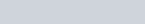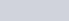If a current of 0.5 ampere flows through a metallic wire for 2 hours,
Question:

If a current of 0.5 ampere flows through a metallic wire for 2 hours, then how many electrons would flow through the wire?

Solution:

= 0.5 A

t = 2 hours = 2 × 60 × 60 s = 7200 s

Thus, Q = It

= 0.5 A × 7200 s

= 3600 C

We know thatnumber of electrons.

Then,

Then,Hence,number of electrons will flow through the wire.